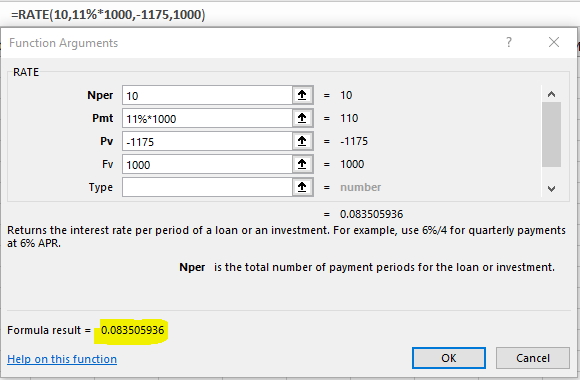# Kempton Enterprises has bonds outstanding with a \$1,000 face value and 10 years left until maturity. They have an 11% annual coupon payment, and their current price is \$1,175. The bonds may be called in 5 years at 109% of face value (Call price = \$1,090).What is the yield to maturity? Do not round intermediate calculations. Round your answer to two decimal places.  %What is the yield to call if they are called in 5 years? Do not round intermediate calculations. Round your answer to two decimal places.  %

Question
13 views

Kempton Enterprises has bonds outstanding with a \$1,000 face value and 10 years left until maturity. They have an 11% annual coupon payment, and their current price is \$1,175. The bonds may be called in 5 years at 109% of face value (Call price = \$1,090).

1. What is the yield to maturity? Do not round intermediate calculations. Round your answer to two decimal places.

%

2. What is the yield to call if they are called in 5 years? Do not round intermediate calculations. Round your answer to two decimal places.

%

check_circle

Calculate the yield to maturity as follows:

MS-Excel --> Formulas --> Financials --> RateTherefore, the yield to maturity is 8.35%.

...

### Want to see the full answer?

See Solution

#### Want to see this answer and more?

Solutions are written by subject experts who are available 24/7. Questions are typically answered within 1 hour.*

See Solution
*Response times may vary by subject and question.
Tagged in